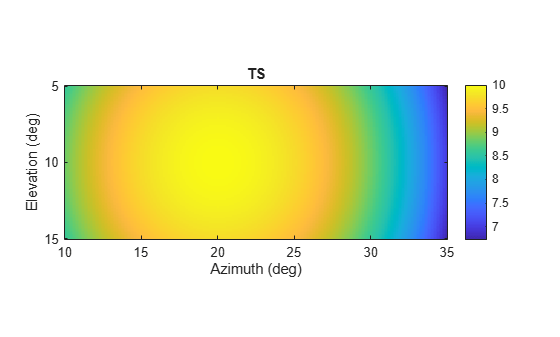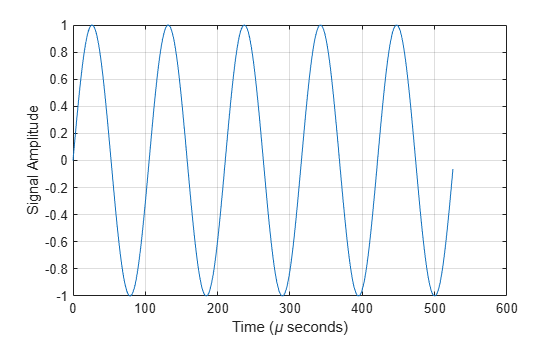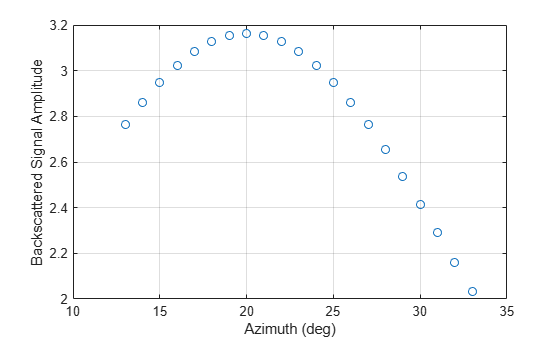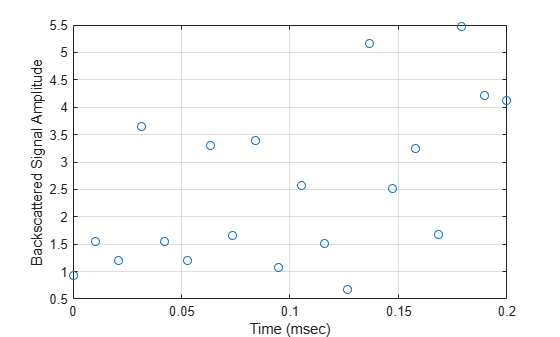Main Content

# phased.BackscatterSonarTarget

Sonar target backscatter

## Description

The phased.BackscatterSonarTarget System object™ models the backscattering of a signal from an underwater or surface target. Backscattering is a special case of sonar target scattering when the incident and reflected angles are the same. This type of scattering applies to monostatic sonar configurations. The sonar target strength (TS) determines the backscattering response of a target to an incoming signal. This object lets you specify an angle-dependent sonar target strength model that covers a range of incident angles.

The object lets you specify the target strength as an array of values at discrete azimuth and elevation points. The object interpolates values for incident angles between array points.

You can employ one of four Swerling models to generate random fluctuations in the target strength. Choose the fluctuation model using the Model property. Then, use the SeedSource and Seed properties to control the fluctuations.

To model a backscattered reflected sonar signal:

1. Define and set up your sonar target. You can set phased.BackscatterSonarTarget System object properties at construction time or leave them to their default values. See Construction. Some properties that you set at construction time can be changed later. These properties are tunable.

2. To compute the reflected signal, call the step method of phased.BackscatterSonarTarget. The output of the method depends on the properties of the phased.BackscatterSonarTarget System object. You can change tunable properties at any time.

Note

Instead of using the step method to perform the operation defined by the System object, you can call the object with arguments, as if it were a function. For example, y = step(obj,x) and y = obj(x) perform equivalent operations.

## Construction

target = phased.BackscatterSonarTarget creates a backscatter sonar target System object, target.

target = phased.BackscatterSonarTarget(Name,Value) creates a backscatter sonar target System object, target, with each specified property Name set to the specified Value. You can specify additional name and value pair arguments in any order as (Name1,Value1,...,NameN,ValueN).

## Properties

expand all

Target strength azimuth angles, specified as a real-valued 1-by-P row vector or P-by-1 column vector. These angles define the azimuth coordinates of each column of the matrix specified by the TSPattern property. P must be greater than two. Angle units are in degrees.

Example: [-45:0.1:45]

Data Types: double

Target strength elevation angles, specified as a real-valued 1-by-Q row vector or Q-by-1 column vector. These angles define the elevation coordinates of each row of the matrix specified by the TSPattern property. Q must be greater than two. Angle units are in degrees.

Example: [-30:0.1:30]

Data Types: double

Sonar target strength (TS) pattern, specified as a real-valued Q-by-P matrix or Q-by-P-by-M array. Q is the length of the vector in the ElevationAngles property. P is the length of the vector in the AzimuthAngles property. M is the number of target patterns. The number of patterns corresponds to the number of signals passed into the step method. You can, however, use a single pattern to model multiple signals reflecting from a single target. Pattern units are dB.

You can also specify the pattern as a function only of azimuth for a single elevation. In this case, specify the pattern as either a 1-by-P vector or an M-by-P matrix. Each row is a separate pattern.

Example: [1,2;3,4]

Data Types: double

Target fluctuation model, specified as 'Nonfluctuating', 'Swerling1', 'Swerling2', 'Swerling3', or 'Swerling4'. If you set this property to a value other than 'Nonfluctuating', use the update input argument when calling the step method.

Example: 'Swerling3'

Data Types: char

Seed source of random number generator for TS fluctuation model, specified as 'Auto' or 'Property'. When you set this property to 'Auto', the System object generates random numbers using the default MATLAB® random number generator. When you set this property to 'Property', you specify the random number generator seed using the Seed property. This property applies when you set the Model property to'Swerling1', 'Swerling2', 'Swerling3', or 'Swerling4'. When you use this object with Parallel Computing Toolbox™ software, you set this property to 'Auto'.

Example: 'Property'

Data Types: char

Random number generator seed, specified as a nonnegative integer less than 232.

Example: 32301

#### Dependencies

To enable this property, set the SeedSource property to 'Property'.

Data Types: double

## Methods

 reset Reset states of System object step Backscatter incoming sonar signal
Common to All System Objects
release

Allow System object property value changes

## Examples

collapse all

Calculate the reflected sonar signal from a nonfluctuating point target with a peak target strength (TS) of 10.0 db. For illustrative purposes, use a simplified expression for the TS pattern of a target. Real TS patterns are more complicated. The TS pattern covers a range of angles from 10° to 30° in azimuth and from 5° to 15° in elevation. The TS peaks at 20° azimuth and 10° elevation. Assume that the sonar operating frequency is 10 kHz and that the signal is a sinusoid at 9500 kHz.

Create and plot the TS pattern.

azmax = 20.0; elmax = 10.0; azpatangs = [10.0:0.1:35.0]; elpatangs = [5.0:0.1:15.0]; tspattern = 10.0*cosd(4*(elpatangs - elmax))'*cosd(4*(azpatangs - azmax)); tspatterndb = 10*log10(tspattern); imagesc(azpatangs,elpatangs,tspatterndb) colorbar axis image axis tight title('TS') xlabel('Azimuth (deg)') ylabel('Elevation (deg)')Generate and plot 50 samples of the sonar signal.

freq = 9.5e3; fs = 100*freq; nsamp = 500; t = [0:(nsamp-1)]'/fs; sig = sin(2*pi*freq*t); plot(t*1e6,sig) xlabel('Time (\mu seconds)') ylabel('Signal Amplitude') gridCreate the phased.BackscatterSonarTarget System object™.

target = phased.BackscatterSonarTarget('Model','Nonfluctuating', ... 'AzimuthAngles',azpatangs,'ElevationAngles',elpatangs, ... 'TSPattern',tspattern);

For a sequence of different azimuth incident angles (at constant elevation angle), plot the maximum scattered signal amplitude.

az0 = 13.0; el = 10.0; naz = 20; az = az0 + [0:1:20]; naz = length(az); ss = zeros(1,naz); for k = 1:naz y = target(sig,[az(k);el]); ss(k) = max(abs(y)); end plot(az,ss,'o') xlabel('Azimuth (deg)') ylabel('Backscattered Signal Amplitude') gridCalculate the reflected sonar signal from a Swerling2 fluctuating point target with a peak target strength (TS) of 10.0 db. For illustrative purposes, use a simplified expression for the TS pattern of a target. Real TS patterns are more complicated. The TS pattern covers a range of angles from 10°to 30° in azimuth and from 5° ro 15° in elevation. The TS peaks at 20° azimuth and 10° elevation. Assume that the sonar operating frequency is 10 kHz and that the signal is a sinusoid at 9500 kHz.

Create and plot the TS pattern.

azmax = 20.0; elmax = 10.0; azpatangs = [10.0:0.1:35.0]; elpatangs = [5.0:0.1:15.0]; tspattern = 10.0*cosd(4*(elpatangs - elmax))'*cosd(4*(azpatangs - azmax)); tspatterndb = 10*log10(tspattern); imagesc(azpatangs,elpatangs,tspatterndb) colorbar axis image axis tight title('TS') xlabel('Azimuth (deg)') ylabel('Elevation (deg)')Generate the sonar signal.

freq = 9.5e3; fs = 10*freq; nsamp = 50; t = [0:(nsamp-1)]'/fs; sig = sin(2*pi*freq*t);

Create the phased.BackscatterSonarTarget System object™.

target = phased.BackscatterSonarTarget('Model','Nonfluctuating',... 'AzimuthAngles',azpatangs,'ElevationAngles',elpatangs,... 'TSPattern',tspattern,'Model','Swerling2');

Compute and plot the fluctuating signal amplitude for 20 time steps.

az = 20.0; el = 10.0; nsteps = 20; ss = zeros(1,nsteps); for k = 1:nsteps y = target(sig,[az;el],true); ss(k) = max(abs(y)); end plot([0:(nsteps-1)]*1000/fs,ss,'o') xlabel('Time (msec)') ylabel('Backscattered Signal Amplitude') grid## More About

expand all

 Urick, R.J. Principles of Underwater Sound, 3rd Edition. New York: Peninsula Publishing, 1996.

 Sherman, C.S. and J.Butler Transducers and Arrays for Underwater Sound. New York: Springer, 2007.

## Support

#### Exploring Hybrid Beamforming Architectures for 5G Systems

Download white paper